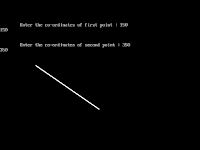Line Drawing using Bresenham Algorithm with patterns such as simple,dash,dash-dot,thick
```# include <stdio .h>
# include <stdio.h>
# include <conio.h>
# include <graphics.h>

void main()
{
int dx,dy,x,y,p,x1,y1,x2,y2;
int gd,gm;

clrscr();

printf("\n\n\tEnter the co-ordinates of first point : ");
scanf("%d %d",&x1,&y1);
printf("\n\n\tEnter the co-ordinates of second point : ");
scanf("%d %d",&x2,&y2);

dx = (x2 - x1);
dy = (y2 - y1);

p = 2 * (dy) - (dx);

x = x1;
y = y1;

detectgraph(&gd,&gm);
initgraph(&gd,&gm,"c:\\tc\\bgi");
putpixel(x,y,WHITE);

while(x <= x2)
{
if(p < 0)
{
x=x+1;
y=y;
p = p + 2 * (dy);
}
else
{
x=x+1;
y=y+1;
p = p + 2 * (dy - dx);
}
putpixel(x,y,WHITE);
}
getch();
closegraph();
}```
Output: